# Example

Suppose you flip a coin 3 times and get heads each time. Is the coin biased?

For most people the answer will be no, but why?

# Binomial Distribution

The binomial distribution is a discrete probability distribution. It is used in situations where you have a repeatable experiment that has 2 outcomes: success or failure. If we know the probability of success is then the binomial distribution can tell us how likely we are to observe successes out of trials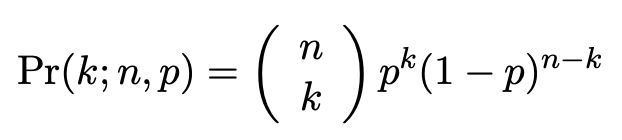The binomial distribution. The first term after the equals sign is called the binomial coefficient.

# Beta Distributions

Beta distributions are continuous probability distributions and be thought of as the opposite of a binomial distribution. If we observe successes in trials, the beta distribution can tell us how likely different values of the success probability are.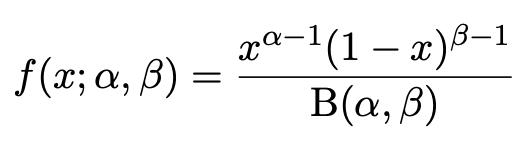The pdf of the beta distribution. B(α, β) is called the beta function and is simply a normalising factor to ensure the total probability is 1.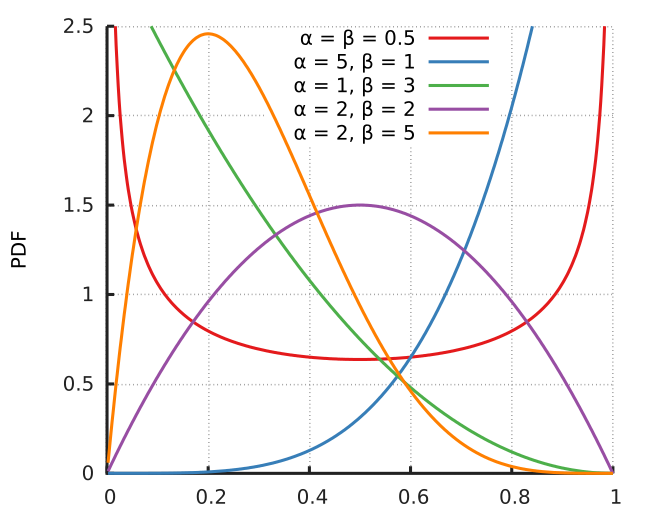The probability density function of the success probability, p, for different values of the parameters α and β. image source

# Conjugate Priors

If we use a beta distribution for our then our is also a beta distribution — this is what is meant by a

# Informed Priors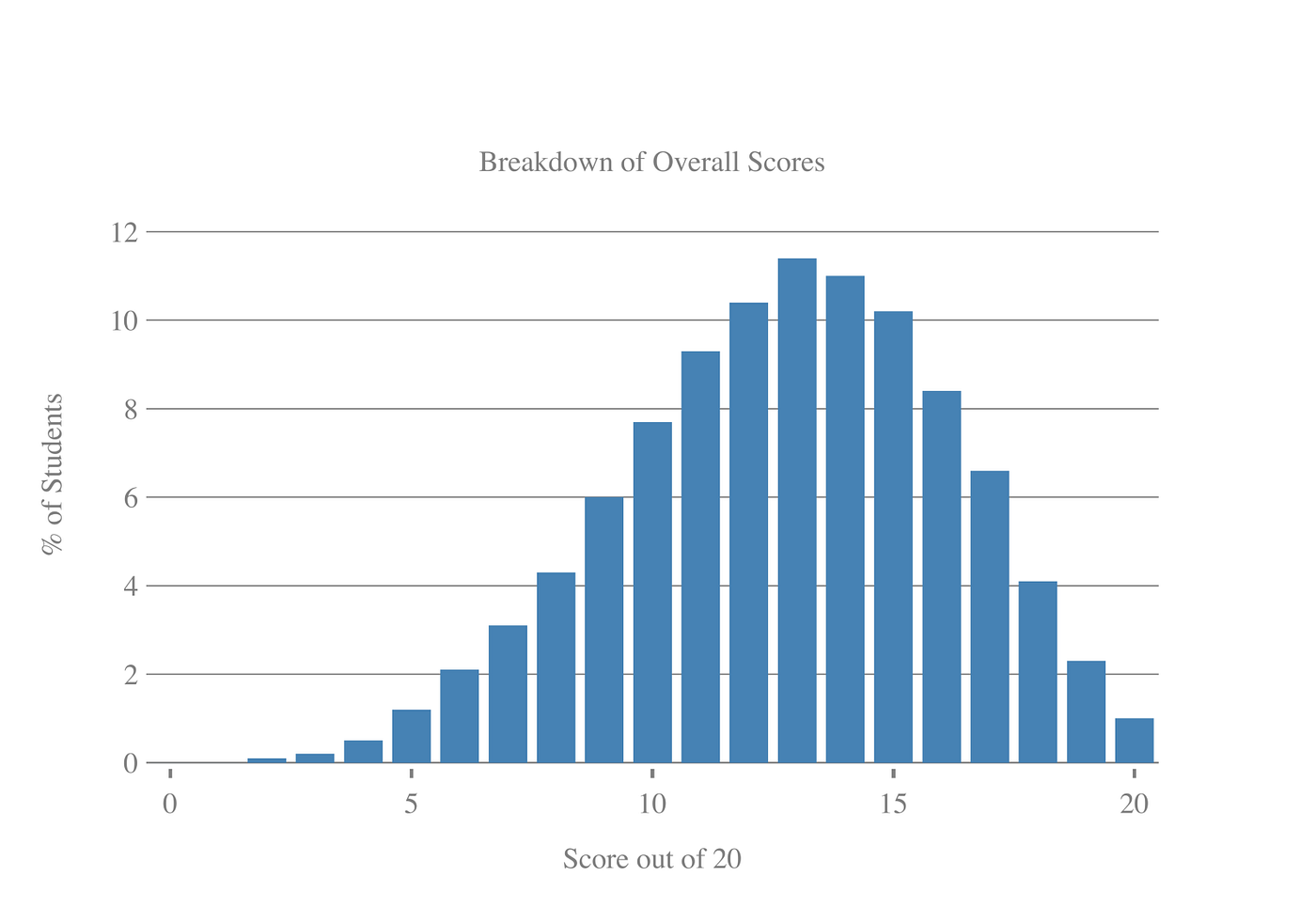Distribution of student scores at end of the quiz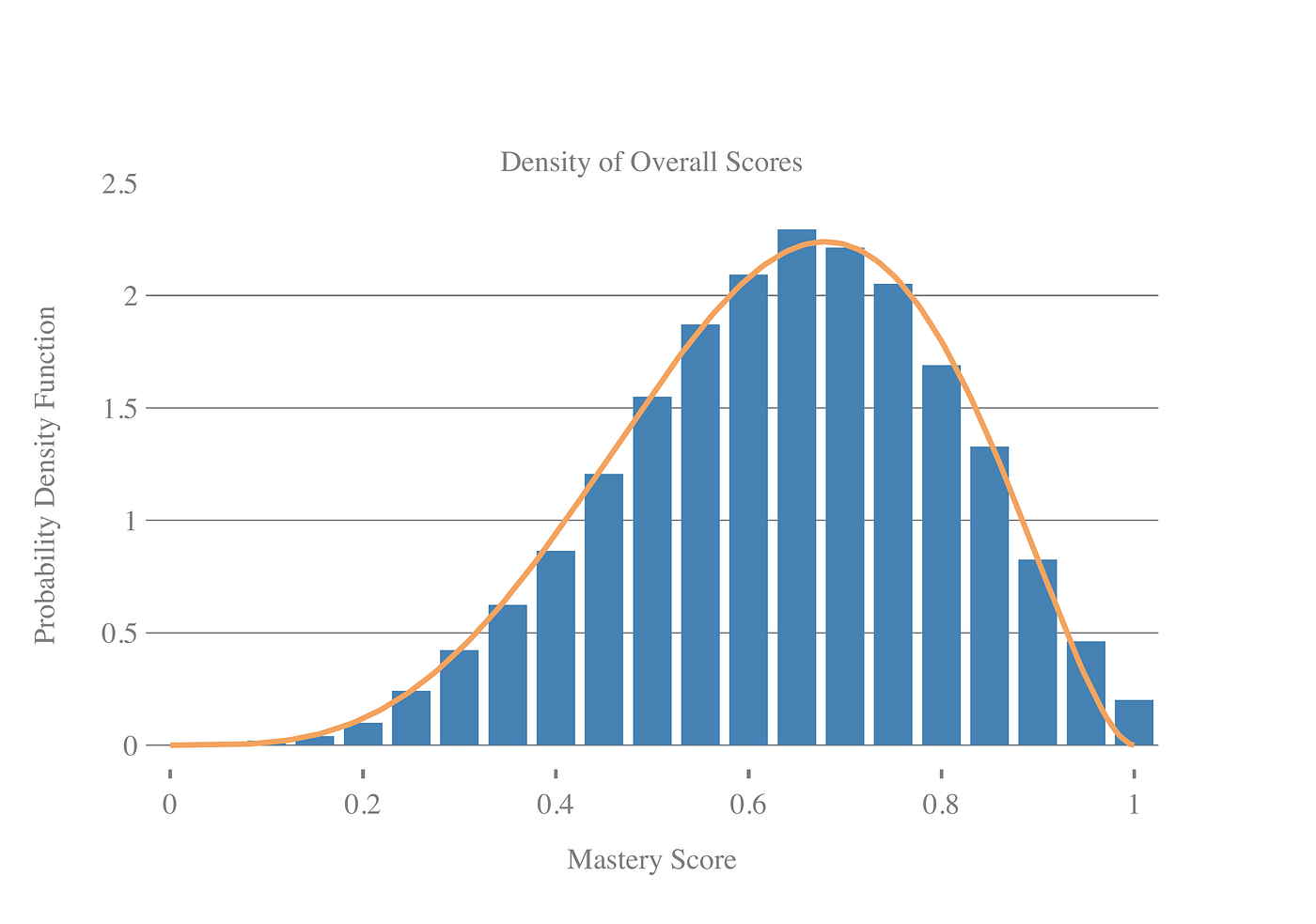Distribution of scores (blue bars) against a beta distribution with parameters α = 4.71 and β = 2.75 (orange line).

# Conclusions

--

--

--

## More from BBC Data Science

Learn more about how the BBC collects, interprets, visualises and democratises data to achieve our goal of putting the audience at the heart of everything we do. https://www.bbc.co.uk/

## Recommended from Medium## What calculators are allowed in GRE?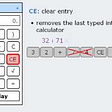## Reasonable Effectiveness Of Mathematics In Natural Sciences## Basic Set Theory by Exercises: Consistency.## What General Relativity Really Means## A Spider-Fly Problem with a Surprising Solution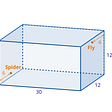## Enumerated Complexity## Matt Crooks

Senior Data Scientist at the TypeForm

## Small-scale initiative in Machine Learning 2021: how did it go?## Introduction to Cooperative Autonomous Multi-Robot Systems## The Missing Library in your Machine Learning Workflow